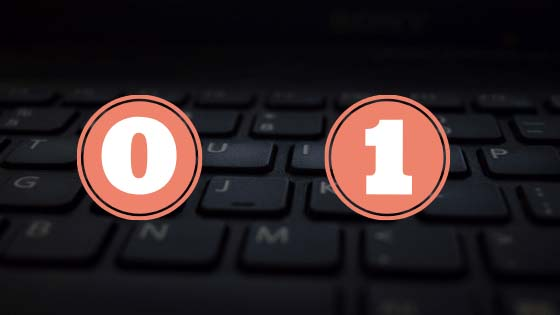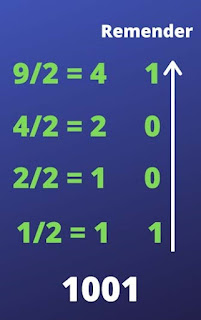The binary number system is a number system that works with only 0 and 1. and this number system is used by only computers. because computers only know the ones and zeros. so it's the base of number 2.binary number system

## What is a Binary number system?

The binary number system is a number system that represents the mathematical numbers in zero (0) and one (1). it has only two symbols 1 for maximum value and 0 for a minimum value of numbers.

for example, if I took the number 9 then the binary representation of number 9 is 1001.

so, how we convert numbers in the binary number.

let's take an example to understand the conversion. let's say we have number 9 which is a math number. so how we can represent 9 in a binary number.

### Method to convert numbers into a binary number.

To convert 9 into a binary number we divide the number 9 by 2. because a binary number is base of 2. as shown in the image given below.So when we divide the 9 by 2. then the reminder we get is 1001. and to convert number 9 into a binary number. we need to arrange the remainder in the bottom to top or right to left manner.

so to arrange the remainder in the bottom to the top manner we get 1001. so this is the binary representation of number 9.

we can also convert a base 2 (binary number) to a decimal number. let's take an example to understand it'.

we have a binary number 1001. so to convert it into its equivalent decimal number we take a single digit of binary number from right to left and multiply with its equivalent base.

D(1001) =
1*23 + 0*22 + 0*21 + 1*2
D(1001) = 8 + 0 + 0 + 1
D(1001) = 9

### Working of Binary number system in Computer

to understand the working of the binary number system in the computer first we need to understand what is bits and bytes.

#### What are Bits and bytes

We all are work with base 10 numbers. and every mathematical condition based on 10 numbers from 0 to 9. but the computer works with bits and bytes.

so the bit is something called 0 or 1. and the 8 bit is equal to 1 byte.



we all have 10 numbers but the computer has only two numbers. a computer has only bits and bytes to work on it. it can be a not(0) or it can be one(1). it cannot be the same at a time. it either be one(1) or either be not(0).

Also, see my other post:

#### How do the Bits work in our hardware?

Our computer is a very complex magnet. and bits are actually magnetic charges. not(0) is a negative charge and one(1) is the positive charge.

by using the electronics computer we can work with base 2, not(0) and one(1)

Our computer is a very delicate magnet. that's why if we used a powerful magnet on our computer. we could potentially store-wide data of hard drive.

we can solve the data flows to our processor. so that's why a bit has worked with two charges negative(0) and positive(1).

Note: it's only because of the stick of a magnet on our computer is not a very good idea.

A bit is the smallest unit in a computer system. it is a basic unit of information in a computer system. using bit we manipulate and store the data in the computer system in memory. the bit is nothing but one or zero.
If I say in the simple form then

Bit = unit of information

Note: if you don't read out our last post then please read it because it will help you to understand what is a binary system and how to use 0 and 1.

#### Conversion of Bit into Byte

Let's say I pick  binary values which are 0 and 1. then how we convert bit to byte is that
One Bit = 1
Two Bits = 01
Three Bits = 100
Eight Bits = 1001 1111 = 1 Byte

Note: here I pick the random values of bits.

Note: but why we need to convert bits in bytes. if you don't know then you must need to read the below lines.

Bits in a row has not real values. bits have only one(1) or not(0) and 1 value at a time. If a bit is 1 or only 0 it would be completely useless to us. the reason why we grouped the bits 0 and 1 is that when we grouped the bits 0 and 1. they represent the base 10 numbers.

## On our calculator, we use only 10 base numbers. and how we add 999+888 and somehow the calculator work with base 2 number how to add these base 10 numbers together and print out the number on the screen add these 999 and 888 we need to use a group of base two numbers. so that's why we need to use a group of base two numbers. and the group of 8 bits is equal to one byte.

We know that there are some types of bits systems.
• 8-bit system.
• 16-bit system.
• 32-bit system.
• 64-bit system.

#### 8-bit system:

In older times we used an 8-bit computer. it means that the computer is only able to process 8 bit at a time. it means we only used 8 once(1) and not(0)  at a single time.

#### 16-bit computer:

In 16 bit computers we only able to process 16 bit at a time. it means we used only 16 once(1) and not(0) at a single time.

#### 32-bit computer:

In a 32-bit computer system we only able to process 32 bit at a time. it means we used only 32 once(1) and not(0) at a single time.

#### 64-bit system:

Now we can work with the 64-bit system. it's means now we can work with 64 bits or 64 once(1) or not(0) at a single time.

If you like this tutorial then please share this post as much as possible to all over the world